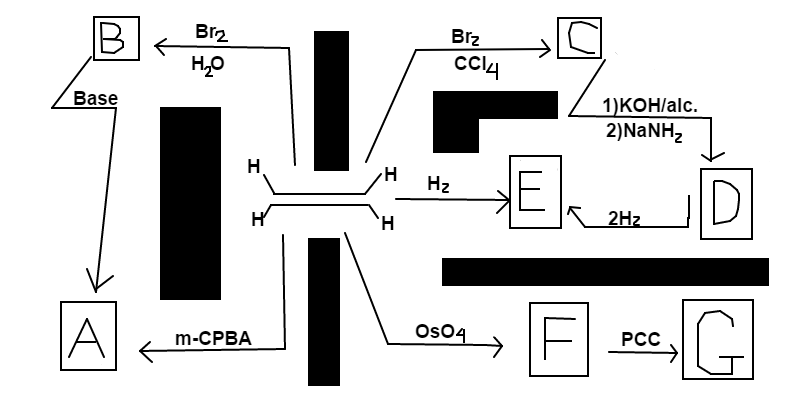# Ethene Reaction Schematic

Chemistry Level 2$Directions:$ Starting with ethene, follow the reaction scheme above. Match each letter with the best major product label represented in the list below. Each label is paired with a number. Your answer should be the number associated with $ABCDEFG$. For example, if $A$ is an simple alkane and $B$ is a diol, the first two digits of your answer will be $12$. $List:$ $1:\text{Simple Alkane} \\ 2:\text{Diol(di-alcohol)} \\ 3:\text{Dial(di-aldehyde)} \\ 4:\text{Alkyne} \\ 5:\text{Halohydrin} \\ 6:\text{Dihalide} \\ 7:\text{Epoxide}$ $Hint:$ Each label is used only once

$Note:$

• alc. stands for alcohol

• PCC stands for Pyridinium Chlorochromate

• m-CPBA stands for meta-Chloro Peroxybenzoic acid

David's Organic Chemistry Set

David's Physical Chemistry Set

×